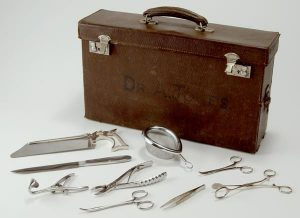Want to share your content on R-bloggers? click here if you have a blog, or here if you don't.If knowledge is power, then knowledge of data.table is something of a super power, at least in the realm of data manipulation in R.

In this exercise set, we will use some of the more obscure functions from the data.table package. The solutions will use set(), inrange(), chmatch(), uniqueN(), tstrsplit(), rowid(), shift(), copy(), address(), setnames() and last(). You are free to use more, as long as they are part of data.table. The objective is to get (more) familiar with these functions and be able to call on them in real-life, giving us fewer reasons to leave the fast and neat data.table universe.

Solutions are available here.

PS. If you are unfamiliar with data.table, we recommend you start with the exercises covering the basics of data.table.

Exercise 1

Load the gapminder data-set from the gapminder package. Save it to an object called “gp” and convert it to a data.table. How many different countries are covered by the data?

Exercise 2

Create a lag term for GDP per capita. That is the value of GDP at the last observation (which are 5 years apart) for each country.

Exercise 3

Using the data.table syntax, calculate the GDP per capita growth from 2002 to 2007 for each country. Extract the one with the highest value for each continent.

Exercise 4

Save the column names in a vector named “temp” and change the name of the year column in “gp” to “anno” (just because); print the temp. Oh my, what just happened? Check the memory address of temp and names(gp), respectively.

Exercise 5

Overwrite “gp” with the original data again. Now make a copy passed by value into temp (before you change the year to anno) so you can keep the original variable names. Check the addresses again. Also, change factors to characters and don’t forget to convert to data.table again.

Exercise 6

A data.table of the number of goals each team in group A made in the FIFA world championship is given below. Import this into R and add a column with the countries’ population in 2017 to the data.table, rounded to the nearest million.

```gA_2014 <- data.table(
country   = c("Brazil", "Mexico", "Croatia", "Cameroon"),
goals2014 = c(7, 4, 6, 1)
)
gA_2014
country goals2014
1:   Brazil         7
2:   Mexico         4
3:  Croatia         6
4: Cameroon         1
```

Exercise 7

Calculate the number of years since the country reached \$8k in GDP per capita at each relevant observation as accurately as the data allows.

Exercise 8

Add a subtly different variable using rowid(). That is the number of the observations among observations where the GDP is below 8k up to and including the given observation. Which country, in each continent, has the most observations above 8k? If there are ties, then list all of the those tied at the top.

Exercise 9

Use inrange() to extract countries that have their life expectancy either below 40 or above 80 in 2002.

Exercise 10

Now, the soccer/football data from exercise 6 came with goals made and goals made against each team as the following:

```gA_2014b <- data.table(
country   = c("Brazil", "Mexico", "Croatia", "Cameroon"),
goals2014 = c("7-2", "4-1", "6-6", "1-9")
)
```

How can you split the goals column into two relevant columns?

(Image by National Museum Wales)